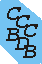Computational Chemistry Comparison and Benchmark DataBase Release 22 (May 2022) Standard Reference Database 101 National Institute of Standards and Technology Home All data for one species Geometry Experimental Calculated Comparisons Bad Calculations Tutorials and Explanations Vibrations Experimental Calculated Scale factors Reactions Entropies Ions List Ions Energy Electron Affinity Proton Affinity Ionization changes point group Experimental One molecule all properties One property a few molecules Geometry Vibrations Energy Electrostatics Reference Data Calculated Energy Optimized Reaction Internal Rotation Orbital Nuclear repulsion energy Correlation Ion Excited State Basis Set Extrapolation Geometry Vibrations Frequencies Zero point energy (ZPE) Scale Factors Bad Calculations Electrostatics Charges Dipole Quadrupole Polarizability Spin Entropy and Heat Capacity Reaction Lookup by property Comparisons Geometry Vibrations Energy Entropy Electrostatics Ion Resources Info on Results Calculations Done Basis functions used I/O files Glossary Conversion Forms Links NIST Links External links Thermochemistry Tutorials Vibrations Entropy Energy Electrostatics Geometry Cost Bad Calculations FAQ Help Units Choose Units Explanations Credits Just show me Summary Using List Recent molecules Molecules Geometry Vibrations Energy Similar molecules Ions, Dipoles, etc. Index of CCCBDB Feedback You are here: Resources > Tutorials > Entropy > Methyl rotor entropy

# How much does a methyl rotor (internal rotation) contribute to the entropy?

The contribution to the entropy for a methyl rotor depends on the reduced moment of inertia and the barrier to internal rotation.

Using ethane (CH3-CH3) as an example we can compute the contribution to entropy of the methyl internal rotation. We will use results calculated at HF/6-31G* and a temperature of 298.15K and determine the contribution to the entropy three different ways:
1. Treating the methyl internal rotation as a harmonic oscillator we have a scaled frequency of 292.6 cm-1. This leads to a contribution to the entropy of 6.1 J K-1 mol-1.
2. Treating the methyl rotation as a free internal rotor with a reduced moment of inertia of 1.55 amu Å2 leads to a contribution to the entropy of 12.0 J K-1 mol-1.
3. The correct treatment as a hindered rotor with a finite barrier of 1044 cm-1 and a reduced moment of inertia of 1.55 amu Å2 (both from a HF/6-31G* calculation) yields 6.8 J K-1 mol-1. This can be summarized for several temperatures in the following table:
Contribution to Entropy for different treatments of the internal rotation in Ethane
100K 298.15K 500K 1000K
Harmonic oscillator 0.7 6.1 10.0 15.6
Free internal rotation 7.5 12.0 14.2 17.1
Hindered rotor 0.7 6.8 11.2 16.1

At low temperatures the harmonic oscillator is a good approximation, and at very high temperatures the free rotor is a better approximation.

The reduced moment of inertia for a methyl group varies depending on the rest of the molecule. In the ethane example it is 1.55 amu Å2. The moment of inertia for a methyl attached to an infinitely heavy mass will be about 3 amu Å2 . For example, in (E)-1,3-pentadiene the methyl rotor reduced moment of inertia is 2.987 amu Å2

See also the discussion on internal rotation in section I.D. A brief description of the thermochemical quantities and methods.